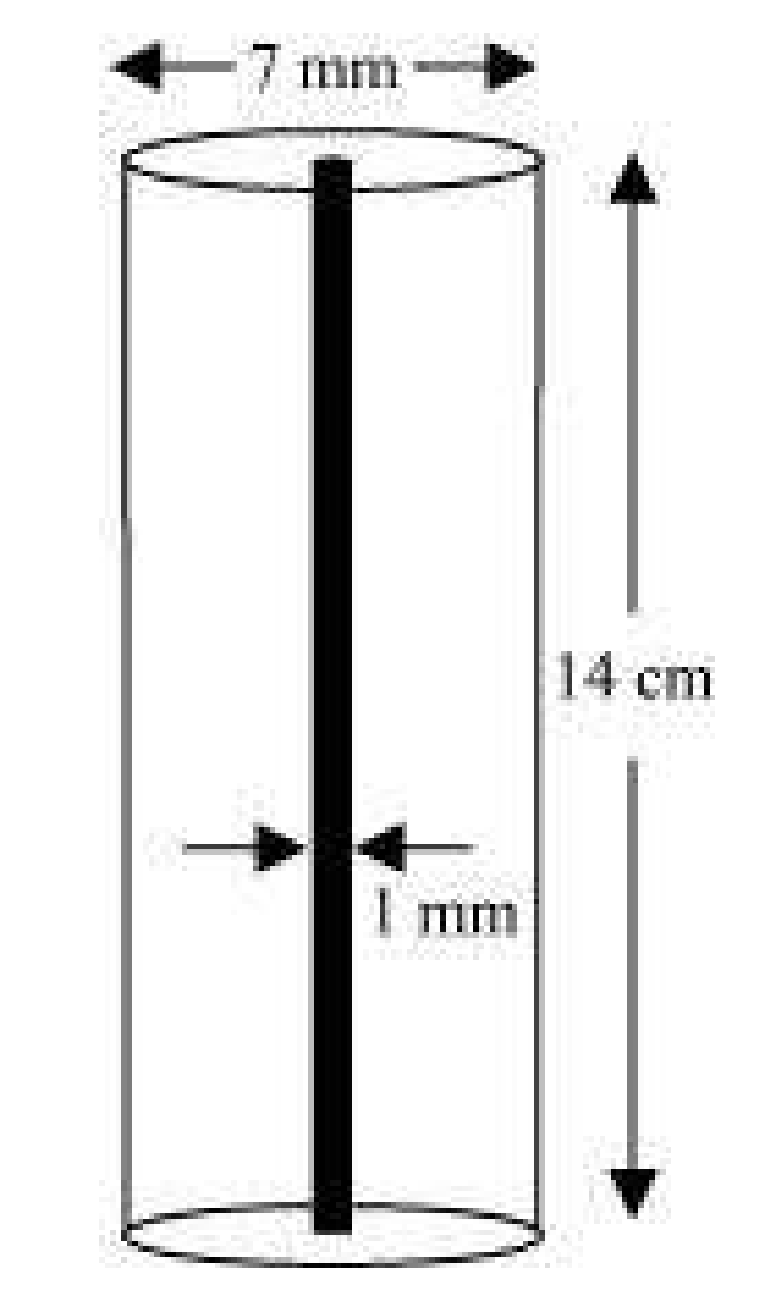Guru

# A lead pencil consists of a cylinder of wood with solid cylinder of graphite filled in the interior. The diameter of the pencil is 7 mm and the diameter of the is 1 mm. If the length of the pencil is 14 cm, find the volume of the wood and that of the graphite. (Assume π = 22/7) Q.7

• 0

What are the different way for solving the question for class 9th question of Surface Areas and Volumes chapter of math of question no.7 of math please suggest me the best way for solving this question of exercise 13.6 A lead pencil consists of a cylinder of wood with solid cylinder of graphite filled in the interior. The diameter of the pencil is 7 mm and the diameter of the is 1 mm. If the length of the pencil is 14 cm, find the volume of the wood and that of the graphite. (Assume π = 22/7)

Share

1.Radius of pencil, r1 = 7/2 mm = 0.7/2 cm = 0.35 cm

Radius of graphite, r2 = 1/2 mm = 0.1/2 cm = 0.05 cm

Height of pencil, h = 14 cm

Formula to find, volume of wood in pencil = (r12-r22)h cubic units

Substitute the values, we have

= [(22/7)×(0.352-0.052)×14]

= 44×0.12

= 5.28

This implies, volume of wood in pencil = 5.28 cm3

Again,

Volume of graphite = r22h cubic units

Substitute the values, we have

= (22/7)×0.052×14

= 44×0.0025

= 0.11

So, the volume of graphite is 0.11 cm3.

• 0